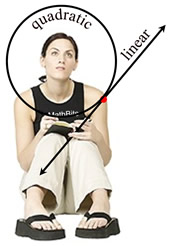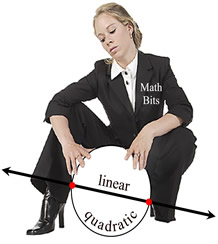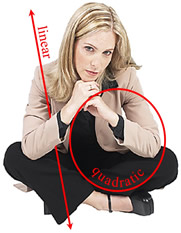Linear Quadratic Systems (line-circle) Terms of Use    Contact Person: Donna RobertsDirections: Grab paper, pencil, and a stratight-edge. You may also want a sheet of graph paper. Beware!! The posed pictures may, or may not, be helpful in solving the problems!
 1. Solve and check this system algebraically:         x2 + y2 = 9      y = 2x - 32. Solve and check this system algebraically:          (x + 1)2 + (y - 1)2 = 18        y = x - 43. Solve and check this system by graphing:         (x - 2)2 + (y - 1)2 = 4        x + y = 14. Solve and check this system by graphing:              x2 + y2 = 4           y + 4 = -x5.
Which ordered pair is a solution to the system shown below?
x2 + y2 = 36   and    y = 6Choose:
 (0,0) (6,0) (0,6) (6,6)

6.
Which choice is the solution set for the system:
x2 + y2 = 13    and    y = -x + 5 ?Choose:
 {(3,2),(3,3)} {(2,3),(3,2)} {(2,3),(2,2)} {(3,2),(2,2)}

7.
Which choice is the solution set for the system:
x
2 + y2 = 25    and    y = x + 1 ?Choose:
 {(3,4),(4,3)} {(3,-4),(3,4)} {(4,3),(-3,4)} {(3,4),(-4,-3)}

8.
How many unique solutions are there to the following system of equations?
(x - 2)2 + (y - 1)2 = 25  and  y = -2x + 15Choose:
 1 2 3 0